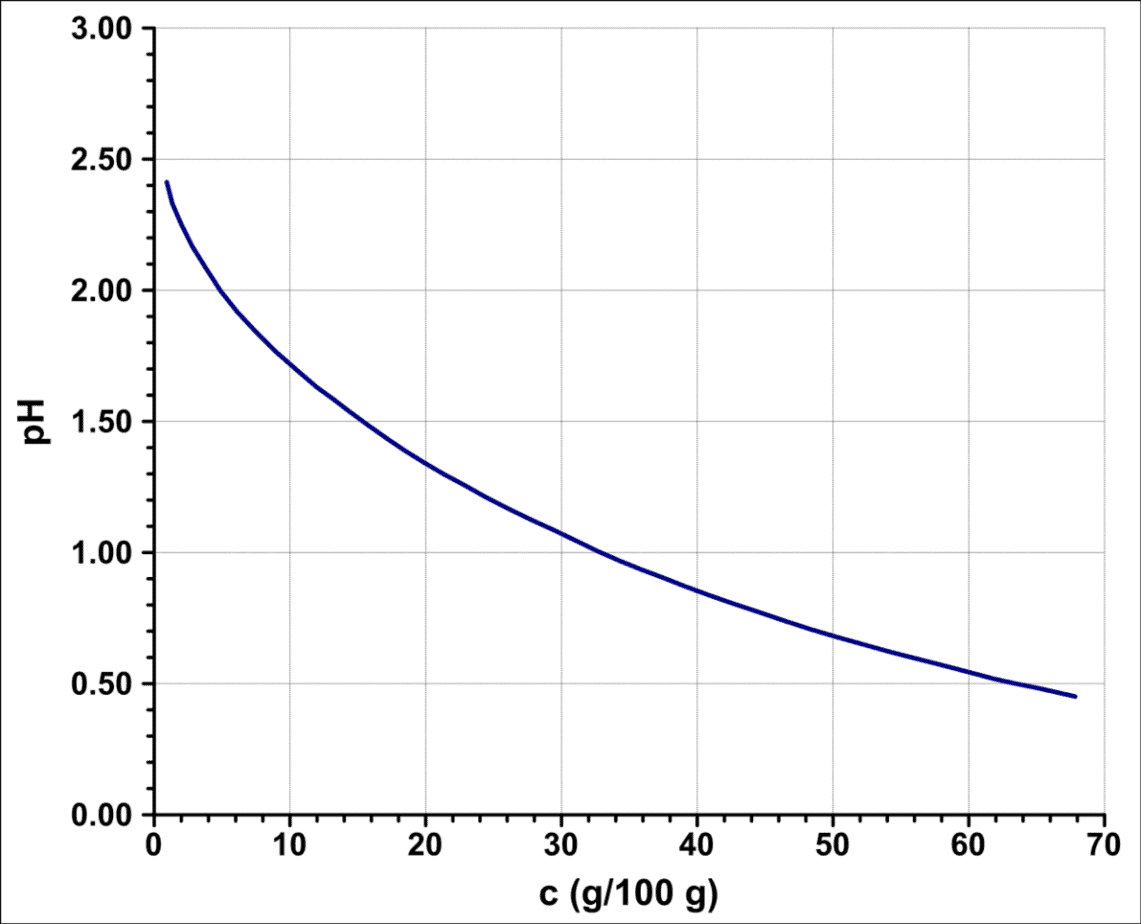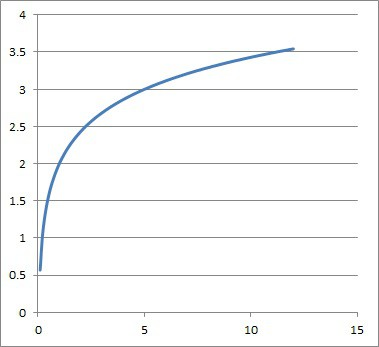# How To Find The Base Of A Logarithm (4 Key Concepts)

Logarithms are used often in math and science (such as chemistry), but they are really just another way to talk about exponents.  Sometimes, we need to solve for the base of a logarithm in an equation or on a graph.

So, how do you find the base of a logarithm?  To find the base of a logarithm from an equation, use the log rules and the formula to convert from logarithm form to exponential form.  To find the base of a logarithm from a graph, find two test points (x and y values) and solve a simultaneous system of equations.

Of course, there are also ways to change the base of a logarithm if you want to use a base of 2, 10, or the natural base e ~ 2.718.

In this article, we’ll talk about how to find the base of a logarithm in an equation or from a graph.  We’ll also talk about the rules of logarithms and how to convert from logarithm form to exponential form.

Let’s get started.

Having math trouble?

Looking for a tutor?

## How To Find The Base Of A Logarithm

To find the base of a logarithm from an equation, it helps to be familiar with the rules of logarithms. Knowing some of the powers of common bases (such as 2, 3, 4, 5, etc.) is also helpful in solving these equations.

Logarithms with bases of 10, 2, and e ~ 2.718 are common, but there is an unlimited supply of bases to choose from. When solving for the base of a logarithm, it helps to know how to convert a logarithmic equation to an exponential equation, so we’ll start with that concept.

### How To Convert Log To Exponential

Remember that a logarithm is just another way of talking about exponents.  In fact, we can convert between the two forms with this relationship:

•  BE = N <–> logB(N) = E  [for B > 0, N > 0, and B not equal to 1]

where B is the base of the logarithm and the exponential, E is the exponent, and N is the number (the argument of the logarithm).In chemistry, the pH of acids and bases follows the graph of a base 10 logarithm. A decrease of 1 in pH means that a substance is 10 times as acidic!

Let’s take a look at some examples of how to convert logarithmic equations to exponential equations.

#### Example 1: Convert Log To Exponential

Let’s say we have the following logarithmic equation:

• log3(x) = 4

In this case, we have B = 3, E = 4, and N = x.

Converting to exponential form, we get:

• 34 = x

Now that we have converted to exponential form, we can solve to get x = 81.

#### Example 2: Convert Exponential To Log

Let’s say we have the following exponential equation:

• 5x = 125

In this case, we have B = 5, E = x, and N = 125.

Converting to logarithmic form, we get:

• log5(125) = x

We can find x = 3 using a calculator and a change of base (more on this later), or we can recognize that 125 is a power of 5: specifically, 53 = 125.

### Log Rules

There are a few rules of logarithms that will make it easier for us to solve logarithmic equations:

• logB(xy) = logB(x) + logB(y)  [logarithm of product equals sum of logarithms of factors]
• logB(x / y) = logB(x) – logB(y)  [logarithm of quotient equals difference of logarithms of numerator and denominator]
• logB(xp) = plog(x)  [logarithm of x to a power of p equals p times the logarithm of x]

Let’s take a look at some examples to make the concepts clear.

## How To Find The Base Of A Logarithmic Equation

To find the base of a logarithmic equation, use the log rules along with the formula to convert logarithms to exponents.

### Example 1: Find The Base Of A Logarithmic Equation

Let’s say we have the following logarithmic equation:

• logB(2) + logB(8) = 4

Note that the two logarithm terms on the left side have the same base of B.  We can use the first logarithm rule listed above to rewrite the left side as:

• logB(2*8) = 4  [log rule: logB(xy) = logB(x) + logB(y)]
• logB(16) = 4

Now we use the formula to convert a logarithmic equation to an exponential equation:

• B4 = 16  [BE = N <–> logB(N) = E]
• B = 2  [16 is 2 raised to the 4th power]

Note that we could also use the third log rule listed above to find B:

• logB(16) = 4
• logB(24) = 4  [16 = 24]
• 4logB(2) = 4  [logB(xp) = plog(x)]
• logB(2) = 1
• B1 = 2
• B = 2

Note that we still had to recognize that 16 = 24.  When working with logarithms, it helps to brush up on your knowledge of powers of 2, 3, 4, 5, etc. so you can recognize them when you see them.

Having math trouble?

Looking for a tutor?

### Example 2: Find The Base Of A Logarithmic Equation

Let’s say we have the following logarithmic equation:

• logB(8x + 16) – logB(x + 2) = 3

Note that the two logarithm terms on the left side have the same base of B.  We can use the second logarithm rule listed above to rewrite the left side as:

• logB((8x + 16) / (x + 2)) = 3  [logB(x / y) = logB(x) – logB(y)]
• logB((8(x + 2)) / (x + 2)) = 3  [8x + 16 = 8(x + 2)]
• logB(8) = 3  [cancel x + 2 in numerator and denominator]

Now we use the formula to convert a logarithmic equation to an exponential equation:

• B3 = 8  [BE = N <–> logB(N) = E]
• B = 2  [since 8 = 23]

## How To Find The Base Of A Log From A Graph

To find the base of a log from a graph, we can take the following steps:

• 1.) Find two test points on the graph
• 2.) Set up two equations: one for each test point.
• 3.) Solve the two equations simultaneously as one system.

Let’s look at an example to see how this would work.

### Example: Find The Base Of A Log From A Graph

Let’s say we have the following graph, with the points (5, 3) and (25, 4).Given the graph of a logarithmic function with some points on the curve, we can find its equation.

If the graph has equation y = logB(x) + K, then the two equations would be:

• 3 = logB(5) + K
• 4 = logB(25) + K

To solve this system, solve both equations for K and set them equal:

• K = K
• 3 – logB(5) = 4 – logB(25)
• logB(25) – logB(5) = 4 – 3  [collect like terms on both sides]
• logB(25 / 5) = 1  [rule of logs: logB(x / y) = logB(x) – logB(y)]
• logB(5) = 1
• B1 = 5
• B = 5

Now that we have found B = 5, we can substitute back into either of the original equations to find K.  We’ll use the first one:

• 3 = logB(5) + K
• 3 = log5(5) + K  [substitute B = 5]
• 3 = 1 + K  [logB(B) = 1 for any B > 0]
• 2 = K

So, our full logarithmic equation from this graph is:

• y = logB(x) + K
• y = log5(x) + 2

## Logarithm Base Change Formula

The logarithm change of base formula allows us to switch from one base B to another.  Here is the logarithm change of base formula:

• logB(N) = logC(N) / logC(B)

### Example 1: How To Convert Ln To Log Base 10

Let’s say we have the logarithmic expression loge(100), and we want to convert it to a logarithm with a base of 10.

In this case, B = e, N = 100, and C = 10.  Using these in the logarithm change of base formula gives us:

• logB(N) = logC(N) / logC(B)
• loge(100) = log10(100) / log10(e)
• loge(100) = 2 / log10(e)  [log10(100) = 2,since 102 = 100]

### Example 2: How To Convert Log To Ln

Let’s say we have the logarithmic expression log10(400), and we want to convert it to a logarithm with a base of e (also known as ln or natural logarithm).

In this case, B = 10, N = 400, and C = e.  Using these in the logarithm change of base formula gives us:

• logB(N) = logC(N) / logC(B)
• log10(400) = loge(400) / loge(10)
• log10(400) = loge(4*10*10) / loge(10)  [since 400 = 4*10*10]
• log10(400) = (loge(4) + loge (10) + loge (10)) / loge(10)  [logB(xy) = logB(x) + logB(y)]
• log10(400) = (loge(4) / loge(10))  + 2

## Conclusion

Now you know how to find the base of a logarithm, either in an equation or from a graph.  You also know how to make a change of base and convert between common logarithms.